Redox

• Oxidation involves the movement of electrons

• This can be monitored using oxidation numbers from AS:

 1) Element = 0 2) Oxide = -2 3) Hydrogen = +1 4) Ionic element =  charge on ion

Redox reactions:

• These are reactions where both oxidation and reduction occur

• Remember:

 Oxidation: Reduction Loss of e Gain of e Ox No increases Ox No decreases Are reducing AGENTS Are oxidizing AGENTS

Constructing redox equations using relevant half - equations

• This is done by balancing the numbers of electrons lost by one half equation with those being gained by another half equation

Example:

• Iron reacts with copper (II) ions forming irone (III) and copper:

Step 1:  Identify the redox half equations:

 Fe à Fe3+ + 3e- Cu2+ + 2e- à Cu

Step 2:  Balance the electrons:

 2Fe à 2Fe3+ + 6e- x2 3Cu2+ + 6e- à 3Cu x3

Step 3:  Add the half equations together and cancel out the electrons:

 2Fe à 2Fe3+ + 6e- 3Cu2+ + 6e- à 3Cu 2Fe + 3Cu2+ + 6e- à 3Cu + 2Fe3+ + 6e-

This gives:

 2Fe + 3Cu2+ à 3Cu + 2Fe3+

Constructing redox equations using oxidation numbers:

• Oxidation numbers can be used as they are a way of keeping track of electrons.
• They can be balanced by following a set of simple rules:

Example:

Hydrogen iodide, HI is oxidised to iodine, I2 by concentrated sulphuric acid, H2SO4, which is reduced to hydrogen sulphide, H2S

Step 1:  Identify the redox half equations:

 HI à 1/2 I2 + e- H2SO4 + 8e- à H2S

Step 2:  Balance the electrons:

 8HI à 4I2 + 8e- x8 H2SO4 + 8e- à H2S

Step 3:  Add the half equations together and cancel out the electrons:

 8HI à 4I2 + 8e- H2SO4 + 8e- à H2S 8HI + H2SO4 + 8e- à 4I2 + H2S + 8e-

Step 4: Balance the oxygen's by adding water to the opposite side:

 8HI + H2SO4 à 4I2 + H2S + 4H2O O 4 0 so add 4 x waters

Step 5: Balance the hydrogen's by adding hydrogen ions to the opposite side:

 8HI + H2SO4 à 4I2 + H2S + 4H2O H 10 10
• Not required in this example

Hints for completing redox reactions:

 In acidic conditions Balance using H+ In alkaline conditions Balance using OH-

Summary:

Step 1:  Identify the redox half equations:

Step 2:  Balance the electrons:

Step 3:  Add the half equations together and cancel out the electrons:

Step 4: Balance the oxygen's by adding water to the opposite side:

Step 5: Balance the hydrogen's by adding hydrogen ions to the opposite side:

 In acidic conditions Balance using H+ In alkaline conditions Balance using OH-

Qu 1-2  P 183

The feasibility of reactions

• At GCSE you predicted weather a reaction would occur based on the reactivity series: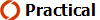The reactivity series (from GCSE)

 Element Oxidised form Reduced form Potassium K+ K Sodium Na+ Na Lithium Li+ Li Calcium Ca2+ Ca Magnesium Mg2+ Mg Aluminium Al3+ Al Zinc Zn2+ Zn Iron Fe2+ Fe Tin Sn2+ Sn Lead Pb2+ Pb (Hydrogen) H+ H Copper Cu2+ Cu Mercury Hg2+ Hg Silver Ag+ Ag Gold Au+ Au
• Those at the top of the reactivity series are so reactive that they prefer to exist in their oxidised form (positive ions).
• Those at the bottom are so unreactive that they prefer to exist in their reduced form (as metal elements).
• Those elements in the middle will do the opposite of whatever they are reacting with:

Consider the following reactions:

A)    Zinc powder is added to a solution of copper sulphate:

• As zinc is more reactive than copper, the reaction proceeds and Zn donates its electrons to Cu2+

Zn(s)         +          Cu2+(aq)            à            Zn2+(aq)       +          Cu(s)

The half equations:

Zn(s)            à     Zn2+(aq)       +          2e-                 Reaction 1

Cu2+(aq)        +       2e-                  à         Cu(s)       Reaction 2

• In reaction 1 the oxidation number of zinc increases from 0 à +2.  Zinc is oxidised.

• In reaction 2 the oxidation number of copper decreases from +2 à 0.  Copper is reduced.

B)    Magnesium powder is added to a solution of zinc sulphate:

• As Magnesium is more reactive than zinc, the reaction proceeds and Mg donates its electrons to Zn2+

Mg(s)     +             Zn2+(aq)         à            Mg2+(s)     +          Zn(s)

The half equations:-

Mg(s)           à     Mg2+(aq)      +          2e-               Reaction 1

Zn2+(aq)         +       2e-                  à         Zn(s)       Reaction 2

• In reaction 1 the oxidation number of magnesium increases from 0 à +2.  Magnesium is oxidised.

• In reaction 2 the oxidation number of zinc decreases from +2 à 0.  Zinc is reduced.

In these 2 examples (A and B) you have seen that zinc has been oxidised and reduced.

• This means that the zinc reactions can be treated as an equilibrium:

 Zn(s)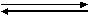Zn2+(aq) + 2e-

Applying Le Chateliers Principle:-

1.     Add electrons to the system and the equilibrium will shift so as to remove electrons.

2.      Remove electrons from the system and the equilibrium will shift so as to produce electrons.

We call this is metal / metal ion system or half cell.

• With a metal whose tendency to loose electrons is greater than that of zinc (Magnesium), the equilibrium will shift towards the reactants (Zn(s))

• With a metal whose tendency to loose electrons is less than that of zinc (Copper), the equilibrium will shift towards the products (Zn2+(aq)).

The electrochemical series:

• The reactivity series is replaced with the electrochemical series:
• Each half cell is given an Eqcell value measured in volts (later)
 Element Oxidised form Reduced form EqcellPotassium K+ K -2.92Sodium Na+ Na -2.71 Lithium Li+ Li -2.59 Calcium Ca2+ Ca -2.44 Magnesium Mg2+ Mg -2.37 Aluminium Al3+ Al -1.66 Zinc Zn2+ Zn -0.76 Iron Fe2+ Fe -0.44 Tin Sn2+ Sn -0.14 Lead Pb2+ Pb -0.13 (Hydrogen) H+ H 0.00 Copper Cu2+ Cu +0.34 Mercury Hg2+ Hg +0.79 Silver Ag+ Ag +0.80 Gold Au+ Au +1.89
• Eqcell values are arranged with the most negative values at the top.

• They are arranged with the highest oxidation number on the left.

• The more negative a value, the greater the tendency for the electrode system to loose electrons.

• This means that the most negative of the 2 systems will move to the LHS whereas the least negative will move to the RHS.

## This means:-

• The systems at the top of the table have a greater tendency to go from right à Left. More negative produces electrons more readily

• The systems at the bottom of the table have a greater tendency to go from left à right.  More positive reacts with the electrons more readily.

Will a reaction actually take place:

Example 1

1)    Write out the half reactions.  The more (-)ve value will produce electrons, the more positive will react with electrons.

 Mg2+(aq) + 2e-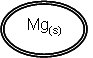Eq = -2.37 1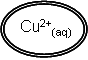+ 2e-Cu(s) Eq = +0.34 2
• From this rule you can easily cirlcle the reactants that you must have in order for the reaction to occurr.

• It is possible to write a reaction from this because this tells you that reaction 1 is going to move to the LHS and reaction 2 is going to move to the RHS.

2)    Draw the direction of each of the half reactions: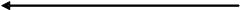Mg2+(aq) + 2e-Eq = -2.37 1+ 2e-Cu(s) Eq = +0.34 2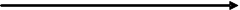3)    Writing balanced redox equation

• Write out the 2 half reactions in the direction they occur.

Mg(s)        à      Mg2+(aq)      +       2e-

Cu2+(aq)       +       2e-          à         Cu(s)

• Balance the electrons (if necessary).

4)    Add the half reactions

• Add the reactions together and the electrons will cancel out:-

Mg(s)        à      Mg2+(aq)      +       2e-

Cu2+(aq)       +       2e-          à         Cu(s)Mg(s)     +    Cu2+(aq)    +    2e-       à         Mg2+(aq)   +    Cu(s)     +    2e-            electrons cancel out

Mg(s)     +    Cu2+(aq)        à      Mg2+(aq)   +    Cu(s)

This can now be extended to metal ion / metal ion systems and also non metal systems:

Example 2:

1)    Write out the half reactions.  The more (-)ve value will produce electrons, the more positive will react with electrons.

 Sn2+(aq) + 2e-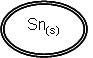Eq = -0.14 1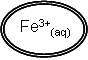+ e-Fe2+(aq) Eq = +0.77 2

2)    Draw the direction of each of the half reactions:Sn2+(aq) + 2e-Eq = -0.14 1+ e-Fe2+(aq) Eq = +0.77 23)    Writing balanced redox equation

• Write out the 2 half reactions in the direction they occur.

Sn(s)        à      Sn2+(aq)      +       2e-

Fe3+(aq)       +       e-          à         Fe2+(aq)                x2

• Balance the electrons.

4)    Add the half reactions

• Add the reactions together and the electrons will cancel out:-

Sn(s)        à      Sn2+(aq)      +       2e-

2Fe3+(aq)       +       2e-          à         2Fe2+(aq)Sn(s)     +    2Fe3+(aq)    +    2e-       à         Sn2+(aq)   +    2Fe2+(aq)     +    2e-            electrons cancel out

Sn(s)     +    2Fe3+(aq)        à      Sn2+(aq)   +    2Fe2+(aq)

Summary steps:

1)    Write out the half reactions.  The more (-)ve value will produce electrons, the more positive will react with electrons.

2)    Draw the direction of each of the half reactions:

3)    Writing balanced redox equation

4)    Add the half reactions

Limitations of predictions using standard electrode potentials:

a)    Effect of changing concentration:

• The symbol q represents standard conditions of 298k and 1 molar solutions.

• Consider the half reaction:

Cu2+(aq)        +       2e-Cu(s)

• Increasing the concentration of the Cu2+ ions moves the equilibrium to the RHS

• This removes electrons from the equilibrium making it less negative

b)    Effect of Eqcell value:

• In practice the Eqcell values have to differ by at least 0.4v in order for the reaction to have enough of a difference in energy to overcome activation energies.

Qu 1  P189

Cells and half cells

Electricity from chemical reactions

• We have seen so far that chemical reactions involve a transfer of electrons from one metal / metal ion system to another.

• It is possible to make these electrons move from one system to another through an external wire - electrical current.

• This is the basis of all batteries.  The chemistry involved is called electrochemistry:

Cells and half cells:

• Batteries are called cells and are based upon the redox reactions studied so far but with the electrons flowing through an external wire.

Zn(s)         +          Cu2+(aq)            à            Zn2+(aq)       +          Cu(s)

• The redox reactions consist of 2 half reactions.  We call these half cells (as 2 of them are needed for a cell)

The half equations:

Zn(s)            à     Zn2+(aq)       +          2e-                 half cell:  flows out of the system to the external wire

Cu2+(aq)        +       2e-                  à         Cu(s)       half cell:  flows into the system from the external wire

Consider the half cell: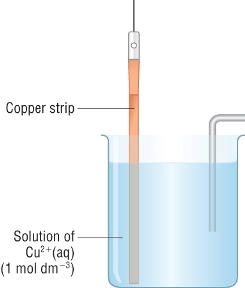Cu2+(aq)        +       2e-Cu(s)                   Ecell = +0.34v   With a more negative half cell: With a half cell that is more negative, the equilibria will move to the RHS.  Electrons move from the more negative to the more positive half cell. With a more positive half cell: With a half cell that is more positive, the equilibria will move to the LHS.  Electrons move from the more negative to the more positive half cell.

So what determines where the copper half cell fits in the electrochemical series?

• It is down to the tendency of a metal to form ions in solution.

• When a metal strip is put into a solution of its ions, an equilibrium is set up:

 M(s)Mz+(aq) + ze-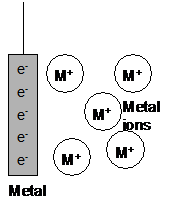As the equilibrium is set up electrons are deposited on the metal foil as metal ions are released into solution. The solution will become positively charged due to the excess metal ions. The metal will become negatively charged as the electrons are deposited upon it. An electrode potential difference is set up between the metal and the ions in solution. Note the overall difference in potential between the ions and the metal is zero.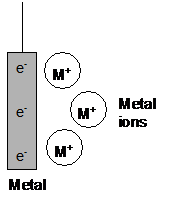The position of the equilibrium between different metals and their ions will be different. This means that the electrode potential differences set up will also be different. These potentials are called absolute potentials and cannot be measured. A reference half cell is used for which all standard electrode potentials are measured against (later).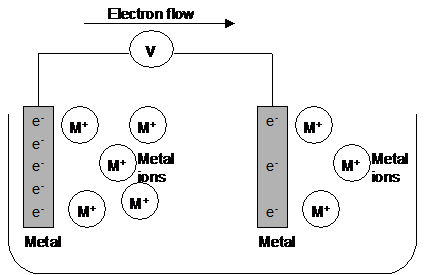By joining 2 of these metal/ion systems together we have a difference in potential between the 2 systems which can be measured.  The potential difference between the 2 metal electrodes is proportional to the difference in the 2 absolute potentials. This is measured by the voltage between the 2 metal electrodes.
• As electrons are removed from one metal/ion system, the equilibrium shifts to replace the electrons.

• As electrons are added to the other metal/ion system, the equilibrium shifts to remove the electrons.

• A continuous flow of electrons occurs until either the metal or ions in the solution run out: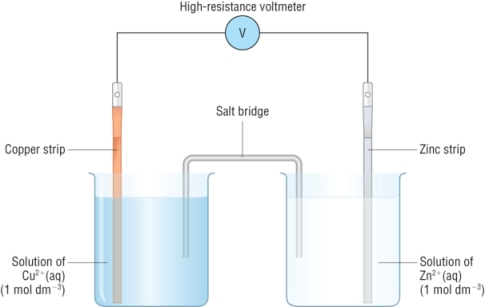Zn(s)     +      Cu2+(aq)         à         Zn2+(aq)     +        Cu(s)   The half equations:   Zn2+(aq)       +          2e-Zn(s)           Most (-)ve half cell - e's flow out of the half cell to the external wire - Equilibrium moves to LHS   Cu2+(aq)        +       2e-Cu(s)         Most (+)ve half cell - e's  flows into the half cell from the external wire - Equilibrium moves to RHS   Current flows in the opposite direction to the flow of electrons
• The salt bridge completes the circuit allowing ions to transfer between the half cells.

• Usually made of filter paper soaked in KNO3

Non - metal / non - metal ion systems:

• A half cell can be made from non metals and their ions:

2H+(aq)          +       2e-H2(g)

 The hydrogen electrode: There is an obvious problem here, there is no metal for the electrons to be transferred from or to the half cell. This is overcome by using a platinum wire coated with finely divided platinum (platinum black), which dips into the acid (H+ ions). A slow stream of hydrogen gas is passed over the platinum black surface, the above equilibrium is gradually set up. Platinum black is porous and retains a lot of hydrogen gas. Platinum metal is also convenient for electron transfer. This is the reference electrode against which all other half cell electrode potential are measured (later)   For this reason the hydrogen electrode has a potential = 0   Conditions: 1M HCl (as the source of H+ions) 1 Atmosphere H2 gas (100KPa) 298 K Inert platinum electrode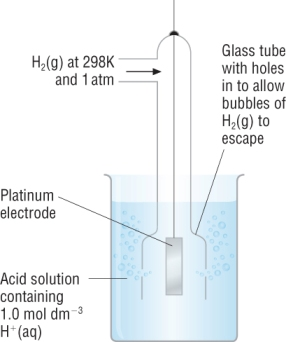Other non - metal / non - metal ion half cells: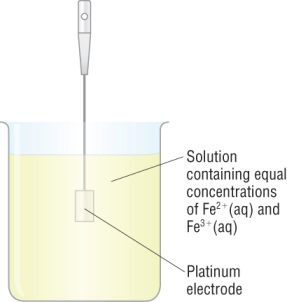Fe2+(aq)        +       e-Fe3+(s)    This system contains iron in its 2 oxidation states. Again there is no metal to allow electron transfer. Platinum is used as an inert electron carrier. Equimolar solutions are used (usually 1M)

Qu 1  P185

Cell potentials

Standard electrode potentials

• These are measured against the reference electrode - The hydrogen half cell

• By defining the hydrogen half cell = 0 all other half cells can be measured.

• This measures its tendency to gain or lose electrons from its half cell with respect to hydrogen

• Remember, the standard electrode potential is measured in volts, emf

Measuring standard electrode potentials: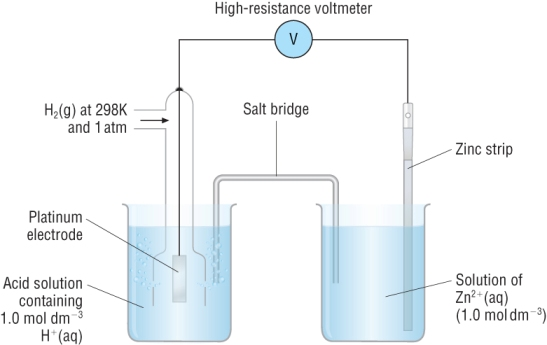• The reading on the voltmeter gives the standard electrode potential of the Zn2+ / Zn half cell = - 0.76

Standard electrode potentials and cell reactions

• Two half cells together give a cell.  This gives a standard cell potential

• The standard cell potential is the difference between the standard electrode potentials of the two half cells.

• The cell reaction is the overall chemical reaction taking place

Example 1:  A magnesium - copper cell is made by connecting 2 half cells together:  Mg2+ / Mg and Cu2+ / Cu

1)    Write out the half reactions.  The more (-)ve value will produce electrons, the more positive will react with electrons.

 Mg2+(aq) + 2e-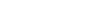Mg(s) Eq = -2.37 1 Cu2+(aq) + 2e-Cu(s) Eq = +0.34 2

2)    Draw the direction of each of the half reactions:Mg2+(aq) + 2e-Eq = -2.37 1+ 2e-Cu(s) Eq = +0.34 2• It is possible to write a reaction from this because this tells you that reaction 1 is going to move to the LHS and reaction 2 is going to move to the RHS.

3.  Balanced chemical equation - Write out the 2 half reactions and the overall equation balancing with electrons

• Write out the 2 half reactions in the direction they occur.

Mg(s)        à      Mg2+(aq)      +       2e-

Cu2+(aq)       +       2e-          à         Cu(s)

• Balance the electrons (if necessary).

4)    Add the half reactions

• Add the reactions together and the electrons will cancel out:-

Mg(s)        à      Mg2+(aq)      +       2e-

Cu2+(aq)       +       2e-          à         Cu(s)                                                    add the half reactionsMg(s)     +    Cu2+(aq)    +    2e-       à         Mg2+(aq)   +    Cu(s)     +    2e-            electrons cancel out

Mg(s)     +    Cu2+(aq)        à      Mg2+(aq)   +    Cu(s)

5)  Calculating emf of electrochemical cells:

Emf   =    Eqpos        -       Eqneg

Emf   =    + 0.34       -        - 2.37

Emf   =    + 2.71v

Rules:

1)    Write out the half reactions.  The more (-)ve value will produce electrons, the more positive will react with electrons.

2)    Draw the direction of each of the half reactions:

3)    Writing balanced redox equation

4)    Add the half reactions

5)    Calculating emf:  Eqcell   =    Eqpos        -       Eqneg

Example 2:  A tin - iron cell is made by connecting 2 half cells together:  Sn2+ / Sn and Fe3+ / Fe2+

1)    Write out the half reactions.  The more (-)ve value will produce electrons, the more positive will react with electrons.

 Sn2+(aq) + 2e-Sn2+(s) Eq = -0.14 1 Fe3+(aq) + e-Fe2+(aq) Eq = +0.77 2

2)    Draw the direction of each of the half reactions:Sn2+(aq) + 2e-Eq = -0.14 1+ e-Fe2+(aq) Eq = +0.77 23.  Balanced chemical equation - Write out the 2 half reactions and the overall equation balancing with electrons

• Write out the 2 half reactions in the direction they occur.

Sn(s)        à      Sn2+(aq)      +       2e-

Fe3+(aq)       +       e-          à         Fe2+(aq)                x2

• Balance the electrons.

4)    Add the half reactions

• Add the reactions together and the electrons will cancel out:-

Sn(s)        à      Sn2+(aq)      +       2e-

2Fe3+(aq)       +       2e-          à         2Fe2+(aq)Sn(s)     +    2Fe3+(aq)    +    2e-       à         Sn2+(aq)   +    2Fe2+(aq)     +    2e-            electrons cancel out

Sn(s)     +    2Fe3+(aq)        à      Sn2+(aq)   +    2Fe2+(aq)

5)    Calculating emf:  Eqcell   =    Eqpos        -       Eqneg

Emf   =    Eqpos        -       Eqneg

Emf   =    + 0.77       -        - 0.14

Emf   =    + 0.91v

Qu 1  P187

Storage and fuel cells

Electrochemical cells

• These are used in every day life as a source of electricity, more commonly known as batteries.

• They all work on the same principle - electrochemistry involving 2 redox reactions

• The most basic cell is the copper / zinc redox system, commonly known as the Daniel cell:The half equations:   Zn2+(aq)   +     2e-Zn(s)           -0.76v      Cu2+(aq)  +     2e-Cu(s)          +0.34v   Overall equation:    Zn(s)     +      Cu2+(aq)         à         Zn2+(aq)     +        Cu(s)   Emf   =    Eqpos        -       Eqneg Emf   =    + 0.34       -        - 0.76 Emf   =    + 1.10v

Modern cells and batteries:

• There are 3 types of electrochemical cells:
 1) Non - rechargable cells: Provides electricity until the chemicals have reacted away. 2) Rechargable cells: The chemicals react providing electricity until they have reacted away.  The difference is that the chemicals can be regenerated by reversing the flow of electrons during charging. 3) Fuel cells: The chemicals react providing electricity but the chemicals needed are constantly supplied.

Fuel cells:

• These have been around for about 150 years but the earliest types didn't produce enough electricity
• This was due to the 2 redox reactions not having a big enough difference to be a viable cell.
• Later ones involve different redox systems to produce enough electricity to be viable for a fuel cell

The hydrogen oxygen fuel cell: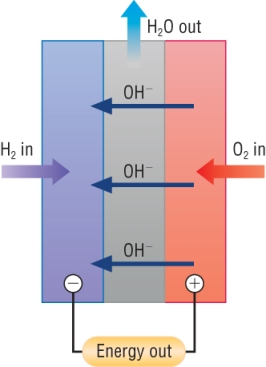The modern fuel cell uses hydrogen and oxygen to create a voltage. The difference is stationary alkaline electrolyte giving a large voltage. The fuel (hydrogen) and oxygen flow into the cell. This produces electricity: The half equations:   2H2O(l)   +     2e-H2(g)    +   2OH-(aq)           -0.83v      1/2O2(g)  +    H2O(l)    +   2e-2OH-(aq)         +0.40v   Overall equation:                       H2(g)     +      1/2O2(g)         à         H2O(l)   Emf   =    Eqpos        -       Eqneg Emf   =    + 0.40       -        - 0.83 Emf   =    + 1.23v The products flow out of the cell.
• Other fuel cells have since been developed based on hydrogen rich fuels - methanol, natural gas and petrol.

Qu 1-2  P191

Hydrogen cells for the future

Development of fuel cell vehicles (FCV's):

• These are based around a hydrogen economy replacing finite oil - based fuels with hydrogen rich fuels.
• Hydrogen rich fuels:  methanol, methane and petrol.
• These are mixed with water and converted to hydrogen using an on board reformer at 250 - 300oC:

CH3OH    +    H2O    à    3H2    +    CO2

• The hydrogen is then used as a fuel source.

Methanol as an alternative to hydrogen:

Advantages:

1)  Liquids are easier to store than gases

2)  Methanol can be produced from biomass

Problems:

1)  Only generate a small amount of power

2)  Produce CO2

Advantages of fuel cell vehicles:

1)  Less CO2 produced

2)  Normal hydrocarbons produce CO which needs to be removed by catalytic converters

3)  Fuel cells are about 40 - 60% efficient / hydrocarbons are about 20%

Storage of hydrogen

• As a gas it is very difficult to store in a tank like liquids.
• Strategies are being developed for the storage of hydrogen:
 Under pressure Adsorbed onto a solid surface Absorbed within a solid Hydrogen can be stored as a liquid under pressure.  Very low temperatures are required to keep them as liquids.  A large 'thermos flask' would be needed to prevent it from boiling. Like a catalyst holds molecules in place.  This means that the hydrogen molecules occupy a smaller volume then as a gas. Similar to before, the hydrogen molecules absorb into the material meaning that the hydrogen occupies a smaller volume.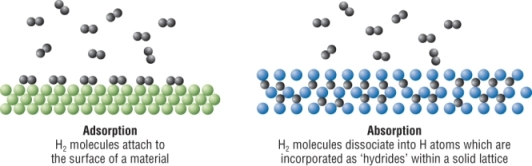Limitations of hydrogen fuel cells:

• Large scale storage and transportation.
• Feasibility of storing a pressurised liquid.
• Current adsorbers and absorbers have a limited lifespan.
• Fuel cells have a limited lifespan.
• Toxic chemicals used in the production of fuel cells.

The hydrogen economy:

• Could contribute to energy needs.
• Limitations need to be overcome.
• Hydrogen is an energy carrier - it is produced from other fuels / wind, solar could generate it as a renewable source.

Qu 1 - 2  P193  /  Qu 1 - 7  P195  /  Qu 1 - 6  P196 - 197